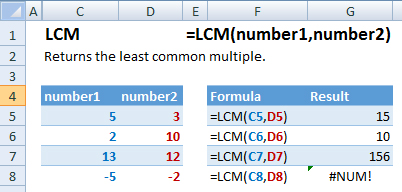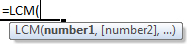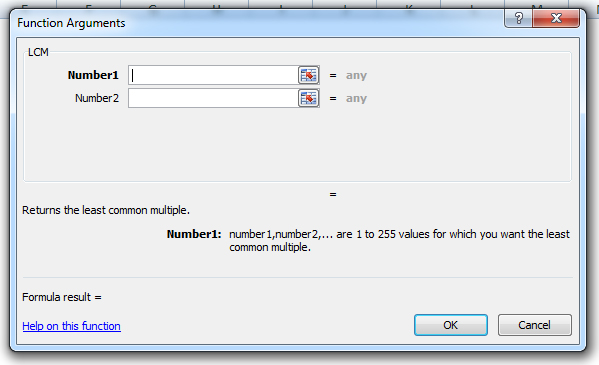### LCM Function Examples – Excel, VBA, & Google Sheets

This Excel Tutorial demonstrates how to use the Excel LCM Function in Excel to calculate the least common multiple, with formula examples.

## LCM Function Description:

The LCM Function Calculates the least common multiple between two or more numbers. The least common multiple is the smallest integer that can be divided by all the numbers provided.

## Formula Examples:Example Formula Result
1 =LCM(C5,D5) 15
2 =LCM(C6,D6) 10
3 =LCM(C7,D7) 156
4 =LCM(C8,D8) #NUM!
AutoMacro - VBA Code Generator

## Syntax and Arguments:

The Syntax for the LCM Formula is:

`=LCM(number1,number2)`

### Function Arguments ( Inputs ):

number1 – Two or more numbers, separated by commas, or an array of numbers.

Use the LCM Function to calculate the least common multiple for a number. Simply enter any number directly into the formula, or reference a cell containing a number.

## LCM Examples in VBAYou can also use the LCM function in VBA. Type:
`Application.Worksheetfunction.Lcm(number1,number2)`
For the function arguments (number1, etc.), you can either enter them directly into the function, or define variables to use instead.

## How to use the LCM Function in Excel:

To use the AND Excel Worksheet Function, type the following into a cell:
`=AND(`
After entering it in the cell, notice how the AND formula inputs appear below the cell:You will need to enter these inputs into the function. The function inputs are covered in more detail in the next section. However, if you ever need more help with the function, after typing “=LCM(” into a cell, without leaving the cell, use the shortcut CTRL + A (A for Arguments) to open the “Insert Function Dialog Box” for detailed instructions: